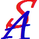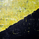27765 views
27765
This is a simple scalping strategy that works for all time frames... I have only tested it on FOREX

It works by checking if the price is currently in an uptrend and if it crosses the 20 EMA .

If it crosses the 20 EMA and its in and uptrend it will post a BUY SIGNAL.
If it crosses the 20 EMA and its in and down it will post a SELL SIGNAL.

The red line is the highest close of the previous 8 bars --- This is resistance
The green line is the lowest close of the previous 8 bars -- This is support
```study(title="Tony's EMA Scalper - Buy / Sell", shorttitle="TUX EMA Scalper", overlay=true)
len = input(20, minval=1, title="Length")
src = input(close, title="Source")
out = ema(src, len)
plot(out, title="EMA", color=blue)
last8h = highest(close, 8)
lastl8 = lowest(close, 8)

plot(last8h, color=red, linewidth=2)
plot(lastl8, color=green, linewidth=2)

bearish = cross(close,out) == 1 and close > close
bullish = cross(close,out) == 1 and close < close

plotshape(bearish, color=red, style=shape.arrowdown, text="Sell", location=location.abovebar)
```Amirfx216
@Amirfx216, You would need to do something using the alertcondition code.

For this it would look like this: